#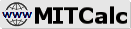Involute gearing - theory

## Involute gearing - theory

This part contains a summary of some theoretical information and formulas related to the geometry design, calculation of force and performance parameters as well as the strength control of involute gearing. These data were used in the calculations below.

• Spur gearing external
• Spur gearing internal
• Planetary Gearing with Spur and Helical Toothing
• Spur gear - Rack
• #### Content:

1. Geometry, dimensions
2. Moment, power, forces, efficiency
3. Planetary Gearing
4. Spur gear - Rack
5. Stress and safety ISO 6336:2006
6. Stress and safety ANSI/AGMA 2001-D04

## 1. Geometry, dimensions.

### Applied formulas (calculation of gearing geometry).

Some of the most important formulas for calculation of the gearing geometry are specified below. In the formulas, indexes 1 and 2 are used for a pinion and a wheel (which is a pair: a sun and a planet, or a planet and a ring gear). For the internal gearing (ring gear), a negative value for the number of internal gear teeth is used, i.e. also a negative value for the centre distance and diameters.

In the case of planetary gearing, the individual gears are dependent on each other and the gearing must be handled as a whole, including the respective constraining conditions (see below).

Basic profile parameters: mn (module, DP for inch calculation), a (pressure angle), ha*, c*, rf* (cutting tool parameters)

Pinion and wheel parameters: z1, z2 (number of teeth of pinion and wheel), x1, x2 (addendum modification), b (helix angle), b (width of gearing)

1. Transmission ratio
i = z2 / z1
2. Module
mt = mn / cos(b) ... Transverse
3. Circular pitch
p = p • mn ... Circular pitch
pt = p / cos(b) ... Transverse circular pitch
ptb = pt • cos(at) ... Base circular pitch
4. Pressure angle
at = arctg(tg(a) / cos(b)) ... Transverse pressure angle
awn = arcinv(2 • (x1 + x2) / (z1 + z2) • tg(a) + inv(a)) ... Pressure angle at the pitch cylinder
awt = arcinv(2 • (x1 + x2) / (z1 + z2) • tg(a) + inv(at)) ... Transverse pressure angle at the pitch cylinder
5. Base helix angle
bb = arcsin(sin(b) • cos(a))
6. Diameter
d = z • mt ... Reference diameter
db = d • cos
(at) ... Base diameter
dw = d • cos
(at) / cos(awt) ... Operating pitch diameter
da = mn • (z / cos
(b) + 2 • (ha* + x - DY) ... Tip diameter
df = d - hf ... Root diameter
7. Center distance
a = (z1 + z2) • mn / (2 • cos
(b)) ... Center distance (pitch)
av = a + (x1 + x2) • mn ... Center distance (production)
aw = a • cos
(at) / cos(atw)  ... Center distance (working)
DY = (a - aw) / mn + (x1 + x2) ... Unit correction
8. Contact ratio
ea = ((da1^2 - db1^2)^0.5 + (da2^2 - db2^2)^0.5 - 2 • aw • sin(awt)) / (2 • p • cos(at) / cos(b)) ... Transverse contact ratio
eb = b / mn / p • sin(b) ... Transverse overlap ratio
eg = ea + eb ... Total overlap ratio

### Principle of corrections, use of corrections.

Approaching and withdrawal of the production tool from the gear center changes the shapes and therefore also properties of the involute toothing. This creates corrected toothing. The illustration shows:

1. Production tool
2. Produced gear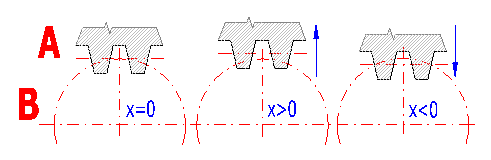Correction (addendum modification) of toothing enables:

• Achieve the exact axis distance.
• Prevent undercutting of teeth (roots of a small number of teeth might be undercut; this decreases the coefficient of duration of the meshing and reduces loading capacity of the teeth)
• Eliminate sharpness of teeth
• Prevent creation of production and operational interference of teeth
• Improve the contact ratio (achieve a contact ratio >1)
• Reduce noise and vibrations of the gearing
• Improve efficiency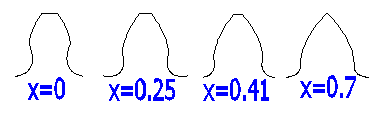Example of a tooth profile (z=10, a=20;b=0), where at X=0 the teeth are undercut and the value x=0.7 causes sharpness of teeth.

#### Recommended values - optimization.

When determining values of corrections, first it is necessary to fulfill functional requirements for toothing, where the most important items include

• Desired axis distance (given sum of both corrections)
• Elimination of teeth undercutting
• Elimination of teeth sharpness

After securing function requirements, it is possible to further optimize corrections in order to improve one or more important toothing parameters. From the frequently used optimizing methods, it is possible to optimize the toothing in order to balance specific slips [5.14 - 5.17] and minimize specific slips [5.18]. For other optimizing processes there is a wide range of recommendations in professional literature, namely the so-called diagrams (charts) of limit corrections, providing a clear view of possibilities and selection of corrections.

## 2. Moment, power, forces, efficiency

### Moment, power, forces in gearing

Torsional moment
Mk [Nm] = Pw * 9550 / n  .............. (SI units)
T [lb.in] = PP * 63000 * / n  ........... (Imperial)
Pw, PP ... power [kW, HP]
n ........... speed [/min]

Power (Spur gear - Rack)
Pw = Ft * v / 1000
Ft ... Tangential force
v .... Rack speed

#### Forces calculation:

Tangential force
Ft = Mk * 2000 / dw
dw ... Operating pitch diameter
MK ... Torsional moment

Axial force
Fa = Ft * tan(b) / cos(awt)
b ...  Base helix angle
awt ... Transverse pressure angle at the pitch cylinder

Fr = Ft * tan(awt)

Normal force
Fn = (Ft2 + Fa2 + Fr2)0.5

Bending moment
Mo = Fa * (dw / 2000)

### Efficiency and losses.

The losses in planetary gearing can be divided into losses due to idle run and losses due to loading. The losses due to idle run (lubrication, unloaded mesh, bearings) are difficult to specify analytically and in general, they are significantly lower than losses due to loading. The losses due to loading arise during power transmission and include:

#### Losses in gearing

The loss coefficient can be approximately described according to the formula:
Spur gearing: zz = 0.5 • f • pe • (1/z1 +- 1/z2)
Helical gearing: zz = 0.25 / cos(b) • f • pe • (1/z1 +- 1/z2)
with:
z1, z2 - number of teeth
f - friction coefficient (0.04 - 0.08)
e - mesh ratio
b - helix angle
Plus sign (+) for external gearing, (-) for internal gearing.

#### Losses in bearings

The loss output can be determined from the relation:
PVL = w • F • f • r
with:
w - angular velocity
f - friction coefficient (0.001 - 0.005)

###### Note: The friction coefficient (for both gearing and bearing) is estimated in the calculation based on the selected accuracy level (gearing roughness) and used lubricant.

For the calculation of loss (efficiency) of the planet gearing, we will use the losses in the helical gearing (carrier stopped) with:

with:
zz0/z1 - loss coefficient sun - planet
zz1/z2 - loss coefficient planet - ring gear

z0,z2 - number of teeth of the sun and ring gear

For individual cases of output flow, it applies for the loss calculation:

 z = ir • zr / (ir - 1) Sun => Planet (carrier) z = zr Sun => Ring gear z = ir • zr / (ir - 1 + zr ) Planet (carrier) => Sun z = - zr / (ir - 1) Planet (carrier) => Ring gear z = - zr / (ir - 1 - ir • zr) Ring gear => Planet (carrier) z = zr Ring gear => Sun

## 3. Planetary Gearing

Planetary gearings consist of a system of gearwheels and a carrier. The so-called suns are aligned with the carrier and the central axis of the gear mechanism. The planets are the gearwheels mounted in a pivoting way on the carrier and they mesh with the suns or with each other. The planets may have one, two or more gearings. Two or multi-speed planets have more constructional variants with wider possibilities; however, they are more complex and expensive to manufacture.

You can see an example of a simple planetary gearing with single-speed toothing of the planet below. This basic type of planetary gearing is also handled in complex in this program.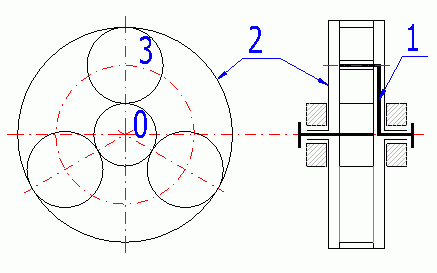##### Simple planetary gearing (differential):

0 - Sun; 1 - Carrier; 2 - Ring gear; 3 - Planet.

If all three members of a simple planetary gearing (0, 1, 2) are free, the system is called a differential (2 degrees of freedom), which enables it to compose / decompose two moves into one. That is used e.g. for machine tools (composition) or for a car differential (move decomposition).

If one of the basic members (0 or 2) is connected to the frame, the system is called planetary gearing (1 degree of freedom), specifically a reductor in case of a drive outwards from the sun or a multiplicator in case of a drive outwards from the carrier. The system with the carrier connected to the frame is called a normal transmission or helical gearing.

Planetary gearings may be arranged in various ways. The most frequent way includes the serial arrangement, where the total transmission ratio (efficiency) is determined by the product of partial transmission ratios (efficiency). The composed gearings often use the possibility to brake individual members, i.e. gear changing.

• Saving of space using an aligned arrangement of the driving and driven shafts
• Lower weight compared to a normal transmission
• High efficiency even at high transmitted outputs
• Compact design

• • Complicated construction, higher requirements for production and assembly accuracy
• • Higher production costs
• • Some limiting conditions (complex assembly)

#### Use:

With respect to the above-specified advantages, the use of planetary gearings is popular in a wide range of applications (e.g. motor vehicle transmissions, building machinery, lifting equipment, marine transmissions, turbine reducers, etc.) The combination of planetary gearing with hydraulic or friction gearing is also common.

### Designing and geometrical relations.

The formulas below use the following indexes.

For:

• Sun - 0
• Planet - 1
• Ring gear - 2

With respect to the possibilities of assembly and functioning of planetary gears, the geometry of the gearwheels cannot be chosen at random. In order to provide proper functioning, the following several conditions must be followed and observed.

#### Condition of alignment.

The planets of the planetary gearings gear with the suns, possibly with other planets. This calculation applies to the joint mesh of a planet with suns (planet, ring gear). As the planet and the ring gear have the same axis, the centre distance between the planet and both suns must be the same.

It applies for the generally corrected wheels that:
aw (0,1) = aw (1,2)
with aw (0,1)=mt • (z0+z1)/2 • COS(alfat)/COS(alfawt(0,1))
with aw (1,2)=mt • (z1+z2)/2 • COS(alfat)/COS(alfawt(1,2))

#### Condition of assembly.

For simple planets and uniform planet distribution, the following condition must be complied with:

g = (abs (z0) + abs (z2))/P
With:
g - must be a random integer
P - number of planets
z - number of teeth

#### Condition of backlash between neighbouring planets.

This condition provides minimum backlash between the planets vmin (1-2 mm, 0.05 in).

Maximum planets P = int(asin((da1+vmin)/(aw • 2)))

## 4. Spur gear - Rack

#### Geometry

This is a standard gearing calculation, where the rack is in gear with the pinion. The manufacturing tool profile can be specified both for the pinion and the gear rack.

It is possible to select the number the pinion teeth, the pressure angle and the tooth slope. Since it has no meaning to correct the gear rack in this case, only the pinion correction is possible (axial distance, gear condition and strength parameter improvement).

In the calculation it is possible to enter the tangential force, which is the force of the gear rack acting on the pinion, and the speed of the gear rack (pinion circumferential speed). These two values are then used to calculate the power and torque transferred via the pinion. Since it is possible to use the gear rack for a range of various design solution, it is then necessary to calculate (estimate) and transfer the transmission requirements on these two values.

#### Strength calculation

Since there are no standards related to the strength calculation of the pinion in gear with the rack, the ISO6336(ANSI/AGMA2001-D04) standard is used for the strength calculation. The gear rack is replaced by a toothed wheel with a high number of teeth (1 000 teeth).

#### Wheel relief coefficient – critical speed

There is no specific methodology of specifying the critical speed for a gear rack application. As a rough estimate, the calculation of two toothed wheels may be used (the gear rack is substituted by a toothed wheel. For a light rack that is not connected to the structure, use the coefficient sR/h=1; if the rack is connected to the structure, use the value of 20.

#### Number of cycles

To determine the lifetime coefficient (YNT, ZNT), a number of cycles must be known. Enter the number of the pinion and gear rack loading cycles.

## 5. Allowable stress and safety for Involute Spur and Helical Gear Teeth  ISO 6336:2006.

In the following paragraphs, the method of the bearing capacity calculation is described. The ISO6336:2006 standard is used for the calculation. The description includes the key formulas used as well as the notes important to understand the calculation and to operate this application. This text does not replace the full text of the standards used.

### ISO 6336-1:2006 Part 1: Basic principles, introduction and general influence factors

This part of ISO 6336 presents the basic principles of, an introduction to, and the general influence factors for, the calculation of the load capacity of spur and helical gears.

#### Basic relations for the gears load

Ft = 2000 * T1,2 / d1,2 = 19098 * 1000 * P / (d1,2 * n1,2) = 1000 * P / v
w1,2 = 2000 * v / d1,2 = n1,2 / 9549

Ft ... (nominal) transverse tangential load at reference cylinder per mesh
T1,2 ...  nominal torque at the pinion (wheel)
d1,2 ... reference diameter of pinion (wheel)
P ... transmitted power
n1,2 ... rotation speed of pinion (wheel)
v ... tangential velocity (without subscript, at the reference circle =tangential velocity at pitch circle)
w1,2 ... angular velocity of pinion (wheel)

#### Application factor KA

The application factor, KA, is used to modify the value of Ft to take into account loads additional to nominal loads, which are imposed, on the gears from external sources. The empirical guidance values in table B.1 ISO 6336-6 can be used (for industry gears and high speed gears).

#### Internal dynamic factor KV

(The internal dynamic factor KV makes allowance for the effects of gear tooth accuracy grade as related to speed and load.

There are three calculation methods (B2006), (C2006) a (C1996).)

The method B is suitable for all the types of spur gears. It is relatively complicated and may give completely unrealistic KV values, if the materials or the degree of accuracy are not properly selected. Therefore it is possible to set the maximum limit for the calculation (pre-set to 5.0). If this limit is exceeded, it is recommended to check the selected material in proportion to the gearing load.

Internal dynamic factor KV(B)
N = n1 / nE1

For N < NS (Subcritical range)
NS = 0.5 + 0.35 * ( Ft * KA / b )0.5 ...... [ Ft * KA / b < 100 ]
NS = 0.85 ...... [ Ft * KA / b >= 100 ]
KV(B) = ( N * K ) + 1
K = ( CV1 * BP ) + ( CV2 * Bf ) + ( CV3 * BK )
BP = c' * fpb eff / ( Ft * KA / b )
Bf  = c' * fta eff / ( Ft * KA / b )
BK = abs (1 + c' * Ca / ( Ft * KA / b ))

For Ns < N < 1.15 (Main resonance range)
KV(B) = ( CV1 * BP ) + ( CV2 * Bf ) + ( CV4 * BK ) + 1

For N >= 1.5 (Supercritical range)
KV(B) = ( CV5 * BP ) + ( CV6 * Bf ) + CV7

For 1.15 < N < 1.5 (Intermediate range)
KV(B) = KV(N=1.5) + ( KV(N=1.15) - KV(N=1.5)) / 0.35 * (1.5 - N)

Coeficients

 1.0 < eg <=2.0 eg > 2.0 CV1 0.32 0.32 CV2 0.34 0.57 / (eg - 0.3) CV3 0.23 0.096 / (eg - 1.56) CV4 0.90 (0.57 - 0.05 * eg ) / (eg - 1.44) CV5 0.47 0.47 CV6 0.47 0.12 / (eg - 1.74) CV7 1.0 < eg <=1.5 0.75 CV7 1.5 < eg <=2.5 0.125 * sin(p * (eg - 1.74)) + 0.875 CV7 eg > 2.5 1.0 Cay1,2 1 / 18 * (sHlim1,2 / 97 - 18.45)2 + 1.5 Cay 0.5 * (Cay1 + Cay2) Ca Ca = Cay

Internal dynamic factor KV(C)

Method C supplies average values which can be used for industrial transmissions and gear systems with similar requirements in the following fields of application:

• subcritical running speed range i.e. (v * z1 / 100) * (u2 / (1 + u2))0.5 < 10 m/s
• external and internal spur gears
• basic rack profile as specified in ISO 53
• straight and helical spur gears with b <= 30
• pinion with relatively low number of teeth z1 < 50
• solid disc wheels or heavy steel gear rim

Method C can also generally be used, with restrictions for the following fields of application:

• all types of cylindrical gears, if (v * z1 / 100) * (u2 / (1 + u2))0.5 < 3 m/s
• For lightweight gear rim and helical gears where b > 30 are values unfavourable (Thus, calculated values tend to be safe).

Method (C2006) is different from (C1996) by adding the coefficient K3.
Example for input values KA * Ft / b = 100; v = 3 m / s; Q = 7 and straight teeth.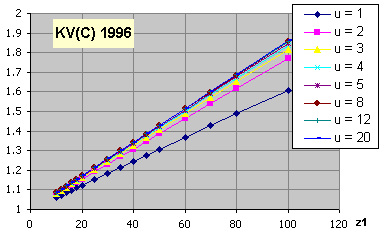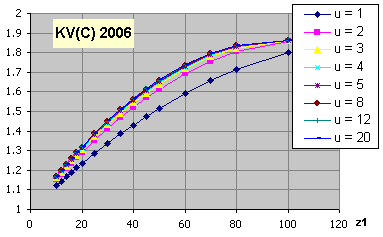KV(C..1996)
KVa,b = 1 + (K1 / ( Ft * KA / b ) + K2) * v * z1 / 100 * (u2 / (1 + u2))0.5 ... [ eb = 0; eb >= 1.0]

KV(C..2006)
KVa,b = 1 + (K1 / ( Ft * KA / b ) + K2) * v * z1 / 100 * K3 * (u2 / (1 + u2))0.5 ... [ eb = 0; eb >= 1.0]

KV = KVa - ea* ( KVa - KVb ) ... [0 < eb < 1.0]

Coeficients

 K1 (Accuracy grades as speclfled in ISO1328-1) K2 3 4 5 6 7 8 9 10 11 12 All Spur gears eb = 0 2.1 3.9 7.5 14.9 26.8 39.1 52.8 76.6 102.6 146.3 0.0193 Helical gears eb >=1.0 1.9 3.5 6.7 13.3 23.9 34.8 47.0 68.2 91.4 130.3 0.0087 SRC = v * z1 / 100 * (u2 / (1 + u2))0.5 K3 = 2.0 ...... [SRC <= 0.2] K3 = -0.357 * SRC + 2.071 ...... [SRC > 0.2]

#### Main resonance of a gear pair NE1

nE1 = 30000 / ( p * z1 ) * ( cga  / mred )0.5

##### where:

mred = m*1 * m*2 / ( m*1 + m*2 )
m*1,2 = J*1,2 / (rb 1,2)2   [kg/mm]
J*1,2 = J1,2 / b1,2
cga = c' * (0.75 * ea + 0.25)
c' = c'th * CM * CR * CB * CE * CFK * cos(b)
c'th = 1 / (0.04723 + 0.15551/zn1 + 0.25791/zn2 - 0.00635*x1 - 0.11654*x1/zn1 - 0.00193*x2 - 0.24188*x2/zn2 + 0.00529*x12 + 0.00182*x22)
c'th = 1 / (0.04723 + 0.15551/zn1  - 0.00635*x1 - 0.11654*x1/zn1 - 0.00193*x2 + 0.00529*x12 + 0.00182*x22) ... for internal gearing
CM = 0.8
CR = 1 + ln(bs / b) / (5 * e(sR/(5 * mn))) ...... [0.2 < bs < 1.2 ]
CB = 0.5 * (CB1 + CB2); CB1,2 = (1 + 0.5 * (1.2 - hf1,2 / mn)) * (1 - 0.02*(20 - aPn))
CE = (( 2 * E1 * E2 ) / ( E1 + E2 )) / 206
CFK = (( Ft * KA / b ) / 100 )0.25...... [ CFK<= 1.0 ]

zn1,2 = z1,2 / cos(b)3

Main resonance of gear with idler gears, inner gears and planet gears are calculated by different process. Details in ISO6336-1.

#### Coefficient KHb (KFb)

This coefficient takes into account the effect of the non-uniform distribution of load over the gear face. Uneven load distribution is caused by an elastic deformation of gears and housing, manufacturing deviations and thermal distortion. Methods, principles and assumptions are given in standard ISO6336-1. Because the determination of the coefficient is dependent on a number of factors and primarily on the specific dimensions and design of the gearbox, is for the design purposes selected the coeficient KHb from graphs based on practical experiences. The calculation is in paragraph .

#### Determination KHb (method C)

Detail description is in ISO6336-1. Here is just a selection of formulas, information and comments that are related to the calculation KHb.

a) KHb = (2 * Fby * cgb / (Fm / b))0.5 ...... [ Fby * cgb / (2 * Fm / b) >= 1.0; KHb >= 2.0 ]
b) KHb = 1 + Fby * cgb / (2 * Fm / b) ...... [ Fby * cgb / (2 * Fm / b)  < 1.0; KHb > 1.0 ]

##### where:

Fm = Ft * KA * KV
Fby =  Fbx *  yb
cgb = 0.85 * cga
b ...... gear width
yb ... Running-in allowance from graph

##### where:

1) Fbx = Choosing your own values
2) Fbx = 1.33 * B1 * fsh + B2 * fma ....... [ Fbx >=  Fbxmin ]
3) Fbx = abs( 1.33 * B1 * fsh - fHb6) ...... [ Fbx >=  Fbxmin ]
4) Fbx = 1.33 * B1 * fsh + fsh2 + fma + fca +fbe

##### where:

B1, B2 coeficients, table 8, ISO6336-1
fHb6 ... Helix slope deviation for Q=6, ISO1328-1
fsh ... Component of equivalent misatignment. It is possible to use several methods (calculation, measurement, estimation). Used formula:
fsh = Fm / b * 0.023 * (abs(B' + K' * l * s / d12 * (d1 / dsh)4 - 0.3) + 0.3) * (b / d1)2 ... [s / l < 0.3]
fsh = Fm / b * 0.046 * (abs(B' + K' * l * s / d12 * (d1 / dsh)4 - 0.3) + 0.3) * (bB / d1)2 ... [s / l < 0.3]
fsh2, fca, fbe ... can be determined by ISO6336-1
B' = 1.0 ... for both spur and single helical gears, for the total transmitted power
K' = arrangement coefficient, gray indicates the less deformed helix of a double helical gear

 K' with stiffening without stiffening A 0.48 0.80 B -0.48 -0.80 C 1.33 1.33 D -0.36 -0.60 E -0.60 -1.00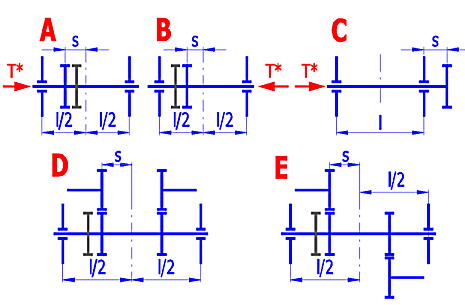l, s .... picture
dsh ... shaft diameter
fma ... mesh misalignment. It is possible to use several methods (calculation, measurement, estimation). Used formula:
fma = (fHb12 + fHb22)0.5 .

#### Determination KHb (Simplified formula)

a) KHb = Acoef * (2 * Fby * cgb / (Fm / b))0.5 ...... [ Fby * cgb / (2 * Fm / b) >= 1.0; KHb > 1.0 ]
b) KHb = Acoef * (1 + Fby * cgb / (2 * Fm / b)) ...... [ Fby * cgb / (2 * Fm / b)  < 1.0; KHb > 1.0 ]

##### where:

Fm = Ft * KA * KV
Fby =  Fb * 0.8 ..... [Fb from ISO 1328]
cgb = 0.85 * cga
b ...... gear width

Acoef = 1.0 ..... Double-sided symmetrically supported gearing
Acoef = (0.9 + 0.15 * (b1 / d1)2 + 0.23 * (b1 / d1)3) ..... Double-sided non-symmetrically supported gearing
Acoef = (0.9 + (b1 / d1)2) ..... Overhung gearing

#### Determination KHb (Approximation from the table)

For the preliminary design is possible to use values ​​from these graphs.
X Axis: Ratio gear width to gear diameter
Y Axis: Factor KHb ..... [min. value = 1.05]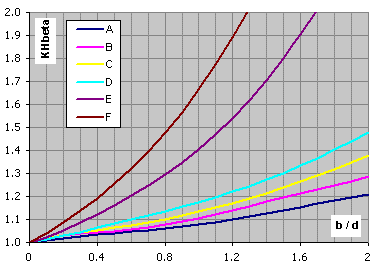Not-hardended gears, VHV<370, design type A-F ... calculation paragraph [2.0]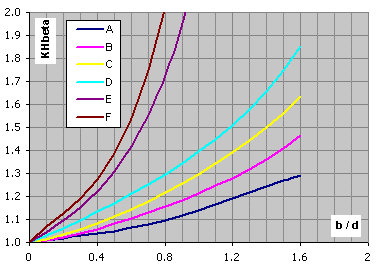Hardended gears, VHV<=370, design type A-F ... calculation paragraph [2.0]

#### Coefficient KFb

KFb = ( KHb )NF
NF = (b / h)2 / (1 + b / h + (b / h)2) ...... [když b / h < 3; pak b / h = 3] ([if b / h < 3; then b / h = 3])

The smaller of the values b1/h1, b2/h2 is to be used as b/h.

#### Coefficient KHa (KFa)

KHa = KFa = eg / 2 * (0.9 + 0.4 * (cga * (fpb - ya)) / (FtH / b)) ...... [eg <= 2.0]
KHa = KFa = 0.9 + 0.4 * (2.0 * (eg - 1.0) / eg)0.5 * cga * (fpb - ya)  / (FtH / b) ...... [eg > 2.0]

Pro: (For:)
KHa > eg / ( ea * Ze2) ...... KHa = eg / ( ea * Ze2)
KHa < 1.0 ...... KHa = 1.0

Pro: (For:)
KFa > eg / (0.25 * ea + 0.75) ...... KFa = eg / (0.25 * ea + 0.75)
KFa < 1.0 ...... KFa = 1.0

fpb = fpt (ISO1328-1)

ya ... Material: St, St(cast), V, V(cast), GGG(perl.), GGG(bai.), GTS(perl.)
ya = fpb * 160 / σHlim [ v < 5m/s ]
ya <= 12800 / σHlim [ 5m/s < v <= 10m/s ]
ya <= 6400 / σHlim [ v > 10m/s ]

ya ... Material: GG, GGG(ferr.)
ya = fpb 0.275 [ v < 5m/s ]
ya <= 22 [ 5m/s < v <= 10m/s ]
ya <= 11 [ v > 10m/s ]

ya ... Material: Eh, IF, NT(nitr.), NV(nitr.), NV(nitrocar.)
ya = fpb 0.075 [ ya <= 3 ]

### ISO 6336-2:2006 Part 2: Calculation of surface durability (pitting)

This part of ISO 6336 specifies the fundamental formulae for use in the determination of the surface load capacity of cylindrical gears with involute external or internal teeth. It includes formulae for all influences on surface durability for which quantitative assessments can be made. lt applies primarily to oil-lubricated transmission, but can also be used to obtain approximate values for (slow-running) grease-lubricated transmissions, as long as sufficient lubricant is present in the mesh at all times.

Safety factor for surface durability (against pitting), SH

Calculate SH separately for pinion and wheel:

SH1,2 =  σHG1,2 /  σH1,2 > SHmin

#### Contact stress σH

σH1 = ZB * σH0 * (KA * KV * KHb * KHa)0.5
σH2 = ZD * σH0 * (KA * KV * KHb * KHa)0.5

The nominal contact stress at the pitch point σH0
σH0 = ZH * ZE * Ze * Zb * (Ft / (b * d1) * (u + 1) / u)0.5

#### Permissible contact stress σHP: Method B

σHP = ZL * ZV * ZR * ZW * ZX * ZNT * σHlim / SHmin = σHG / SHmin

The pitting stress limit σHG
σHG = σHP * SHmin

#### Zone factor ZH

ZH = (2 * cos(bb) * cos(awt) / (cos(at)2 * sin(awt)))0.5

#### Single pair tooth contact factors ZB and ZD

M1 = tan(awt) / ((((da1 / db1)2 - 1.0)0.5 - 2 * p / z1) * (((da2 / db2)2 - 1.0)0.5 - (ea - 1.0) * 2 *  p / z2))0.5
M2 = tan(awt) / ((((da2 / db2)2 - 1.0)0.5 - 2 * p / z2) * (((da1 / db1)2 - 1.0)0.5 - (ea - 1.0) * 2 *  p / z1))0.5

Spur gears with, ea > 1.0
ZB = 1.0 ... [ M1<= 1.0 ]
ZB = M1 .... [ M1 > 1.0 ]
ZD = 1.0 ... [ M2<= 1.0 ]
ZD = M2 .... [ M2 > 1.0 ]

Helical gears with, eb >= 1.0
ZB = ZD = 1.0

Helical gears with, eb < 1.0
ZB = M1 - eb * (M1 - 1.0) ... [ ZB >= 0 ]
ZD = M2 - eb * (M2 - 1.0) ... [ ZD >= 0 ]

(For internal gears, ZD shall be taken as equal to 1.0)

#### Elasticity factor ZE

ZE = (p * ((1.0 - n12) / E1 + (1 - n22) / E2))-0.5

##### where

n1,2 ... Poisson's ratio
E1,2 ... modulus of elasticity

#### Contact ratio factor Ze

Ze = ((4.0 - ea) / 3 * (1.0 - eb) + eb / ea)0.5  ... [ 0 <= eb < 1.0 ]
Ze = (1.0 / ea)0.5  ... [ eb >= 1.0 ]

#### Helix angle factor, Zb

Zb = 1 / (cos(b))0.5

#### Life factor ZNT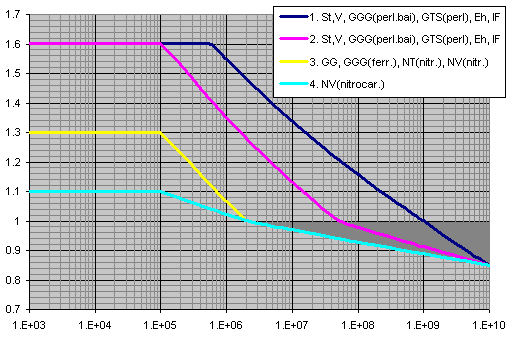X axis ... number of cycles
Y axis ... ZNT

#### Lubricant factor ZL

ZL = CZL + 4 * (1.0 - CZL) / (1.2 + 80 / n50)2 = CZL + 4 * (1.0 - CZL) / (1.2 + 134 / n40)2
CZL = 0.83 ... [ σHlim < 850 ]
CZL = σHlim / 4375 + 0.6357 ... [ 850 <= σHlim <= 1200 ]
CZL = 0.91 ... [ 1200 < σHlim ]
n50 ( n40) ... Nominal viscosity in 50°C (40°C) [mm2/s]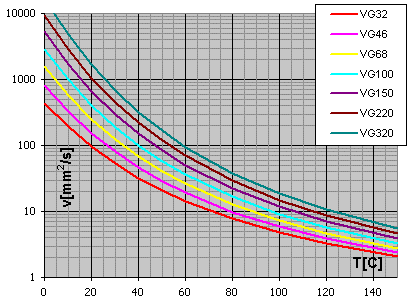Diagram viscosity / temperature for viscosity index VI = 50

#### Velocity factor ZV

ZV = CZV + 2 * (1.0 - CZV) / (0.8 + 32 / v)0.5
CZV = CZL + 0.02

#### Roughness factor, ZR

ZR = (3 / Rz10)CZR
CZR = 0.15 ... [ σHlim < 850 ]
CZR = 0.32 - 0.0002 * σHlim ... [ 850 <= σHlim <= 1200 ]
CZR = 0.08 ... [ 1200 < σHlim ]
Rz10 = Rz * (10 / rred)(1/3)
rred = (r1 * r2) / (r1 + r2)
r1,2 = 0.5 * db1,2 * tan(awt)

#### Work hardening factor, ZW

The work hardening factor, ZW takes account of the increase in the surface durability due to meshing a steel wheel (structural steel, through-hardened steel) with a hardened or substantially harder pinion with smooth tooth flanks.

Pinion Surface-hardened, Gear through-hardened
ZW = 1.2 * (3 / RzH)0.15 ... [ HB < 130 ]
ZW = (1.2 - (HB - 130) / 1700) * (3 / RzH)0.15 ... [ 130 <= HB <= 470 ]
ZW = (3 / RzH)0.15 ... [ HB > 470 ]

ZW for static stress
ZW = 1.05 ... [ HB < 130 ]
ZW = 1.05 - (HB - 130) / 680 ... [ 130 <= HB <= 470 ]
ZW = 1.0 ... [ HB > 470 ]
RzH = Rz1 * (10 / rred)0.33 * (Rz1 / Rz2)0.66) / ( n40 * v / 1500)0.33) ... [ 3 <= RzH <=16 ]

Through-hardened pinion and gear
ZW = 1.0 ... [ HB1/HB2 < 1.2 ]
ZW = 1.0 + A * (u - 1.0) ... [ 1.2 <= HB1/HB2 <= 1.7 ]
ZW = 1.0 + 0.00698 * (u - 1.0) ... [ 1.7 < HB1/HB2 ]
A = 0.00898 * HB1/HB2  - 0.00829

ZW for static stress
ZW = 1.0

### ISO 6336-3:2006 Part 3: Calculation of tooth bending strength.

This part of ISO 6336 specifies the fundamental formulae for use in tooth bending stress calculations for involute external or internal spur and helical gears with a rim thickness sR > 0.5 * ht for external gears and sR >1.75 * mn for internal gears.

Safety factor for bending strength (safety against tooth breakage), SF

Calculate SF separately for pinion and wheel:

SF1,2 =  σFG1,2 /  σF1,2 >= SFmin

#### Tooth root stress σF

σF = σF0 * KA * KV * KFb * KFa

The nominal tooth root stress σF0
σF0 = Ft / (b * mn) * YF * YS * Yb * YB * YDT

#### Permissible bending stress σFP : Method B

σFP = σFlim * YST * YNT * YdrelT * YRrelT * YX / SFmin = σFG / SFmin

Tooth root stress limit σFG
σFG = σFP * SFmin

#### The form factor, YF : Method B

YF = (6 * hFe / mn * cos(aFen)) / ((sFn / mn)2 * cos(an))

#### Tooth root normal chord sFn ; radius of root fillet rF ; bending moment arm hFe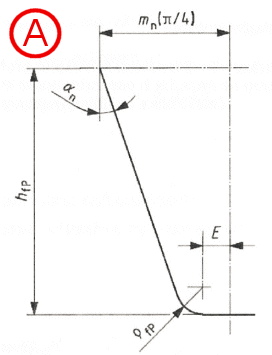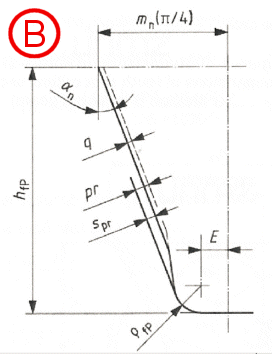Dimensions and basic rack profile of the teeth (finished profile)
A...without undercut
B...with undercut

auxiliary values
E = p / 4 * mn - hfP * tan(an) + spr / cos(an) - (1 - sin(an) * rfP / cos(an)
spr = pr - q
spr = 0 when gears are not undercut
rfPv = rfP ... external gears
rfPv = rfP + mn * (x0 + hfp/mn - rfP/mn)1.95 / (3.156 * 1.036z0) ... internal gears
x0 ... the pinion-cutter shift coefficient
z0 ... the number of teeth of the pinion cutter
G = rfPv / mn - hfP / mn + x
H = 2 / zn * (p / 2 - E / mn) - T
T = p / 3 ... external gears
T = p / 6 ... internal gears
q = 2 * G / zn * tan(q) - H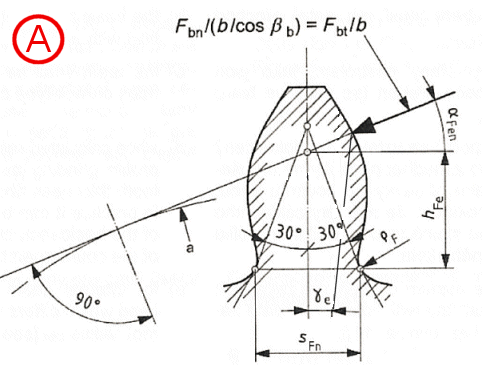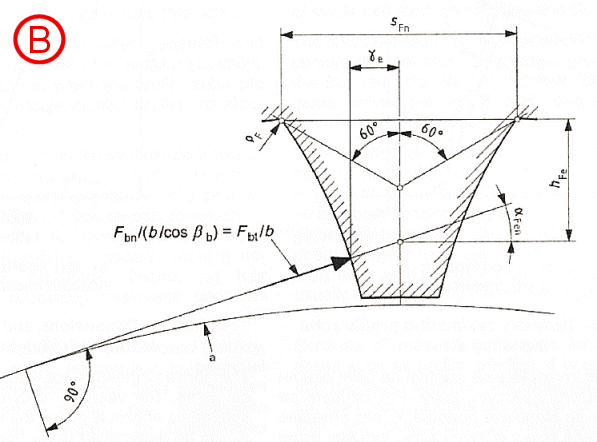Determination of normal chordal dimensions of tooth root critical section for Method B
A...external gears
B...internal gears

Tooth root normal chord sFn
sFn / mn = zn * sin(p/3 - q) + (3)0.5 * (G / cos(q) - rfPv / mn) ... external gears
sFn / mn = zn * sin(p/6 - q) + (G / cos(q) - rfPv / mn) ... internal gears

rF  / mn = rfPv / mn + 2 * G2 / (cos(q) * (zn * cos(q)2 - 2 * G))

Bending moment arm hFe
hFe / mn = 0.5 * ((cos(ge) - sin(ge) * tan(aFen)) * den / mn - zn * cos(p/3 - q) - G / cos(q) + rfPv / mn)) ... external gears

hFe / mn = 0.5 * ((cos(ge) - sin(ge) * tan(aFen)) * den / mn - zn * cos(p/6 - q) - (3)0.5 * (G / cos(q) - rfPv / mn))) ... internal gears

Parameters of virtual gears

bb = arcsin(sin(b) * cos(an))
zn = z / (cos(bb))3
ean= ea / (cos(bb))2
dn = mn * zn
pbn = p * mn * cos(an)
dbn = dn * cos(an)
dan = dn + da - d
den = 2 * z / abs(z) * ((((dan / 2)2 - (dbn / 2)2)0.5 - p * d * cos(b) * cos(an) * (ean - 1) / abs(z))2 + (dbn / 2)2)0.5

*The number of teeth z is positive for external gears and negative for internal gears

aen = arccos(dbn / den)
ge = (0.5 * p + tan(an) * x) / zn + inv(an) - inv(aen)
aFen = aen - ge

#### Stress correction factor YS

The stress correction factor YS is used to convert the nominal tooth root stress to local tooth root stress.
YS = (1.2 + 0.13 * L) * qs(1 / (1.21 + 2.3 / L))
L = SFn / hFe
qs = SFn / (2 * rF)

#### Stress correction factor for gears with notches in fillets YSg

YSg = 1.3 * YS / (1.3 - 0.6 * (tg / rg)0.5) ... [ (tg / rg)0.5 < 2.0 ]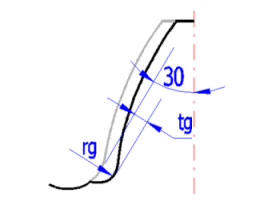#### Helix angle factor Yb

Yb = 1 - eb * b / 120 ... [if b > 30; b = 30]

#### Rim thickness factor YB

external gears
YB = 1.0 ... [sR / ht >= 1.2]
YB = 1.15 * ln(8.324 * mn / sR) ... [0.5 < sR / ht < 1.2]

internal gears
YB = 1.0 ... [sR / mn >= 3.5]
YB = 1.6 * ln(2.242 * ht / sR) ... [1.75 < sR / mn < 3.5]

#### Deep tooth factor YDT

YDT = 1.0 ... [ean <= 2.05] or [accuracy grade > 4]
YDT = -0.666 * ean + 2.366 ... [2.05 < ean <= 2.5] and [accuracy grade <= 4]
YDT = 0.7 ... [ean > 2.5] and [accuracy grade <= 4]

#### Life factor YNT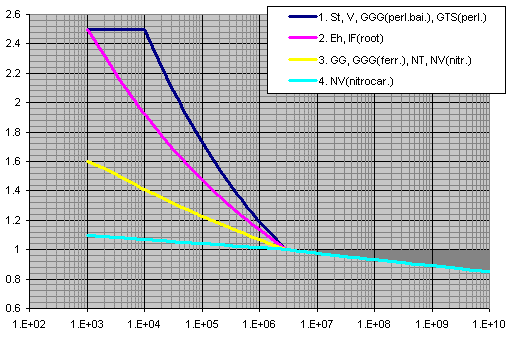X axis ... number of cycles
Y axis ... YNT

#### Relative notch sensitivity factor YdrelT for reference stress

YdrelT = Yd / YdT = (1 + (r' * c*)0.5) / (1 + (r' * cT*)0.5)
c* = cP* * (1 + 2 * qs)
cP* = 1 / 5 = 0.2
cT* = cP* * (1 + 2 * 2.5)

Material: GG [σB=150MPa], GG, GGG(ferr.)[σB=300MPa]
r' = 0.31

Material: NT, NV
r' = 0.1005

Material: St, V, GTS, GGG(perl.), GGG(bai.)
r' = MAX(MIN(13100 / Rp0.2(2.1) - (MAX(600;Rp0.2)-600)(0.35) / 1600;0.32);0.0014)

Material: Eh, IF(root)
r' = 0.003

#### Relative notch sensitivity factor YdrelT for static stress

Material: St, V, GGG(perl.), GGG(bai.)
YdrelT = (1 + 0.82 * (YS - 1) * (300 / σ0.2)(1/4)) / (1 + 0.82 * (300 / σ0.2)(1/4))

Material: Eh, IF, IF(root)
YdrelT = 0.44 * YS + 0.12

Material: NT, NV
YdrelT = 0.20 * YS + 0.60

Material: GTS
YdrelT = 0.075 * YS + 0.85

Material: GG, GGG(ferr.)
YdrelT = 1.0

#### Relative surface factor YRrelT for reference stress

Rz < 1 mm

Material: V, GGG(perl.), GGG(bai.), Eh, IF
YRrelT = 1.12

Material: St
YRrelT = 1.07

Material: GG, GGG(ferr.), NT, NV
YRrelT = 1.025

1mm < Rz < 40 mm

Material: V, GGG(perl.), GGG(bai.), Eh, IF
YRrelT = 1.674 - 0.529 * (Rz + 1)0.1

Material: St
YRrelT = 5.306 - 4.203 * (Rz + 1)0.01

Material: GG, GGG(ferr.), NT, NV
YRrelT = 4.299 - 3.256 * (Rz + 1)0.0058

YRrelT = 1.0

#### Size factor YX

YX = 1.0 ... All materials for static stress

YX ... Material: St, St(cast), V, V(cast), GGG(perl.), GGG(bai.), GTS(perl.)
YX = 1.0 ... [ mn <= 5 ]
YX = 1.03 - 0.006 * mn ... [ 5 < mn < 30 ]
YX = 0.85 ... [ mn >= 30 ]

YX ... Material: Eh, IF(root), NT, NV, NT(nitr.), NV(nitr.), NV(nitrocar.)
YX = 1.0 ... [ mn <= 5 ]
YX = 1.05 - 0.01 * mn ... [ 5 < mn < 25 ]
YX = 0.80 ... [ mn >= 25 ]

YX ... Material: GG, GGG(ferr.)
YX = 1.0 ... [ mn <= 5 ]
YX = 1.075 - 0.015 * mn ... [ 5 < mn < 25 ]
YX = 0.70 ... [ mn >= 25 ]

### ISO 6336-5 Strength and quality of materials.

This part of ISO 6336 describes contact and tooth-root stresses, and gives numerical values for both limit stress numbers. It specifies requirements for material quality and heat treatment and comments on their influences on both limit stress numbers.

The allowable stress numbers, σH lim, and the nominal stress numbers, σF lim, can be calculated by the following equation:

a) σHlim = A * x + B
b) σFlim = A * x + B

where x is the surface hardness HBW or HV and A, B are constants

#### Requirements for material quality and heat treatment

The three material quality grades ML, MQ and ME, stand in relationship to
- ML stands for modest demands on the material quality and on the material heat treatment process during gear manufacture.
- MQ stands for requirements that can be met by experienced manufacturers at moderate cost.
- ME represents requirements that must be realized when a high degree of operating reliability is required

In this calculation, except σHlim and σFlim, are proposed other material parameters that are necessary for calculating the gearing. The values of Ro, E and Poisson constant are commonly available. For the proposal of the tensile strength Rm and yield strength Rp0.2 was used information from the ISO 1265 and specialized literature. Parameters for the time-strength curves were obtained from ISO6336-2 and 3. These curves can be seen in a small graph in the calculation.
All calculated values are design and based on empirical experience. The exact values for a concrete material you can obtain from your manufacturer or from material tests.

###### Hardness notice

Values HB for HB<=450 steel ball, HB>450 carbide ball
Values HB used recalculation HB=HV-HV/20
Values HRC used recalculation  HRC=(100*HV-14500)/(HV+223)

## 6. Allowable stress and safety for Involute Spur and Helical Gear Teeth ANSI/AGMA 2001-D04

### Fundamental Rating Factors and Calculation Methods for Involute Spur and Helical Gear Teeth ANSI/AGMA 2001-D04

#### dynamic factor, Kv

Kv =  (C / (C + vt))−B
C = 50 + 56 * (1.0 − B) ... [ 6 ≤ Av ≤ 12 ]
B = 0.25 * (Av − 5.0)0.667

vt max = [C + (14 − Av)]2

The empirical guidance values from table B.1 ISO 6336-6 are used.

#### Elastic coefficient, Cp

Cp = (1 / p * (((1 - mP2) / EP) + ((1 - mG2) / EG)))0.5  ... [lb/in2]0.5
mP and mG is Poisson’s ratio for pinion and gear, respectively; EP and EG is modulus of elasticity for pinion and gear [lb/in2].

Cf = 1.0

#### Hardness ratio factor, CH

Through hardened gears
CH = 1.0 + A * (mG - 1.0)
A = 0.00898 *(HBP / HBG) - 0.00829
HBP is pinion Brinell hardness number [HB]; HBG is gear Brinell hardness number,[HB].
This equation is valid for the range 1.2 ≤ HBP / HBG ≤ 1.7 For HBP / HBG < 1.2, A = 0.0 HBP / HBG > 1.7, A = 0.00698

Surface hardened/through hardened values
CH = 1.0 + B * (450 - HBG)
B = 0.00075 * (2.71828)-0.0112 * (fp)
fp is surface finish of pinion, microinches, Ra
if fp>64 ... CH = 1.0

Km = f (Cmf, Cmt)
Km = Cmf

#### Face load distribution factor, Cmf

Cmf = 1.0 + Cmc * (Cpf * Cpm + Cma * Ce)
Cmc is 1.0 for gear with unmodified leads; Cmc is 0.8 for gear with leads properly modified by crowning or lead correction.
Cpf = F / (10 * d) − 0.025 ... [F<=1.0]
Cpf = F / (10 * d) − 0.0375 + 0.0125 * F ... [1.0<F<=17.0]
Cpf = F / (10 * d) − 0.1109 + 0.0207 * F − 0.000228 * F2 ... [17.0<F<=40.0]
Cpm = 1.0 ... [S1 / S < 0.175]
Cpm = 1.1 ... [S1 / S >= 0.175]
Cma = A + B * F + C * F2

 A B C 1…Open gearing 0.247 0.0167 -0.0000765 2…Commercial enclosed gearboxes 0.127 0.0158 -0.0001093 3…Precision enclosed gearbox 0.0675 0.0128 -0.0000926 4…Extra precision enclosed gearbox 0.038 0.0102 -0.0000822

Ce = 0.8 ... [gearing is adjusted at assembly; gearing is improved by lapping]
Ce = 1.0 ... [for all other conditions]

#### Reliability factor, KR

KR = 1.50 [Fewer than one failure in 10 000]
KR = 1.25 [Fewer than one failure in 1000]
KR = 1.00 [Fewer than one failure in 100]
KR = 0.85 [Fewer than one failure in 10]
KR = 0.70 [Fewer than one failure in 2]

#### Rim thickness factor, KB

KB = 1.6 * ln(2.242 / mB) ... [for mB<1.2]
KB = 1.0 ... [for mB>=1.2]

mB = tR / ht
tR is gear rimthickness below the tooth root [in]; ht is gear tooth whole depth [in]

#### Pitting resistance

The contact stress number formula for gear teeth is:

sc = Cp (Wt * Ko * Kv * Ks * Km * Cf / (d * F * I))0.5

Allowable contact stress number
The relation of calculated contact stress number to allowable contact stress number is:

sc ≤ (sac * ZN * CH) / (KT * SH * KR)

Pitting resistance power rating
The pitting resistance power rating is:

Pac = (p * np * F / 396 000) * I / (Ko * Kv * Ks * Km * Cf) * ((d * sac * ZN CH) / (Cp * SH * KT * KR))2

#### Safety coefficient for surface durability

SH = sac / sc * (ZN * CH) / (KT * KR)

#### Bending strength

The fundamental formula for bending stress number in a gear tooth is:

st = Wt * Ko * Kv * Ks * (Pd * Km * KB / (F * J))

Allowable bending stress number
The relation of calculated bending stress number to allowable bending stress number is:

st ≤ (sat * YN) / (SF * KT * KR)

Bending strength power rating
The bending strength power rating is:

Pat = (p * np * F / 396 000) * (F * J) / (Ko * Kv * Pd * Ks * Km * KB) * (sat * YN) / (SF * KT * KR)

#### Safety coefficient for bending strength

SF = sat / st * YN / (KT * KR)

Wt = 33000 * P / vt = 2 * T / d = 396000 * P / (p * np * d)

P is transmitted power [hp]; T is transmitted pinion torque [lb*in]; vt is pitch line velocity at operating pitch diameter, [ft/min]
vt = p * np * d / 12

### Allowable stress numbers, sac and sat ANSI / AGMA 2001-D04

This part of ANSI / AGMA 2001-D04 describes the allowable stress numbers sac and sat, for pitting resistance and bending strength.

Allowable stress numbers in this standard are determined or estimated from laboratory tests and accumulated field experiences. They
are based on unity overload factor, 10 million stress cycles, unidirectional loading and 99 percent reliability. For service life other than 10
million cycles, the allowable stress numbers are adjusted by the use of stress cycle factors YN and ZN

The allowable stress numbers sac and sat can be calculated by the following equation:

a) sac = A * x + B
b) sat = A * x + B

where x is the surface hardness HBW and A, B are constants

#### Requirements for material quality and heat treatment.

These requirements are specified in this standard and are divided in three material quality grades 1,2 an 3.

In this calculation, except sac and sat, are proposed other material parameters that are necessary for calculating the gearing. The values of p, E and Poisson constant are commonly available. For the proposal of the tensile strength Rm and yield strength Rp0.2 was used information from the ISO 1265 and specialized literature. All calculated values are design and based on empirical experience. The exact values for a concrete material you can obtain from your manufacturer or from material tests.

###### Hardness notice

Values HB for HB<=450 steel ball, HB>450 carbide ball
Values HB used recalculation HB=HV-HV/20
Values HRC used recalculation  HRC=(100*HV-14500)/(HV+223)

^